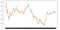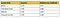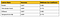# Machine Learning Mini-Project: Predicting Stock Closing Prices from Intraday Stock Prices on the NIFTY index.

## DATASmoothed and Raw Time Series

## MODEL SELECTION

`rocket = MiniRocket(random_state = 2468)  trainx_transform = rocket.fit_transform(Xtrain_sktime)valx_transform = rocket.transform(Xtest_sktime)clf = RidgeClassifierCV(alphas = np.logspace(-4,4, num = 100), normalize = True)clf.fit(trainx_transform, ytrain_sktime)predicted = clf.predict(valx_transform)print("Accuracy with Rocket: %2.3f" % accuracy_score(ytest_sktime, predicted))print("Matthews CC:%2.3f" % matthews_corrcoef(ytest_sktime, predicted))`
`steps = [("extract",RandomIntervalFeatureExtractor(n_intervals = "sqrt",                features=[np.mean, np.std, _slope])),("clf", DecisionTreeClassifier())]time_series_tree = Pipeline(steps)tsf = TimeSeriesForestClassifier(estimator=time_series_tree,n_estimators = 100,criterion = "entropy",bootstrap=True,oob_score=True,random_state = 2222,n_jobs=-1)tsf.fit(Xtrain_sktime, ytrain_sktime)print("Accuracy:  ", accuracy_score(ytest_sktime, tsf.predict(Xtest_sktime)))print("MCC:  ", matthews_corrcoef(ytest_sktime, tsf.predict(Xtest_sktime)))`
`i = Input(shape=(trainx.shape[-2], 1))m = TCN()(i)m = Dense(1, activation = 'sigmoid')(m)early_stopping = EarlyStopping(patience = 50, restore_best_weights=True, min_delta = 0.000)reduceLR = ReduceLROnPlateau(factor = 0.5, patience = 5, min_delta = 0.01)\from keras.optimizers import *model = Model(inputs=[i], outputs=[m])model.summary()model.compile(loss = "binary_crossentropy", optimizer = Adam(lr = 1e-3))model.reset_states()model.fit(trainx, trainy,validation_data = (valx, y_test),shuffle = True,callbacks = [early_stopping, reduceLR],batch_size = 64,epochs = 200)`

## MODEL EVALUATION-4 HOUR WINDOWResults for the 4 hour window.

## MODEL EVALUATION-5 HOUR WINDOWModel performance for 5 hour window

## CONCLUSION

Machine Learning Engineer

## More from Peijin Chen

Machine Learning Engineer